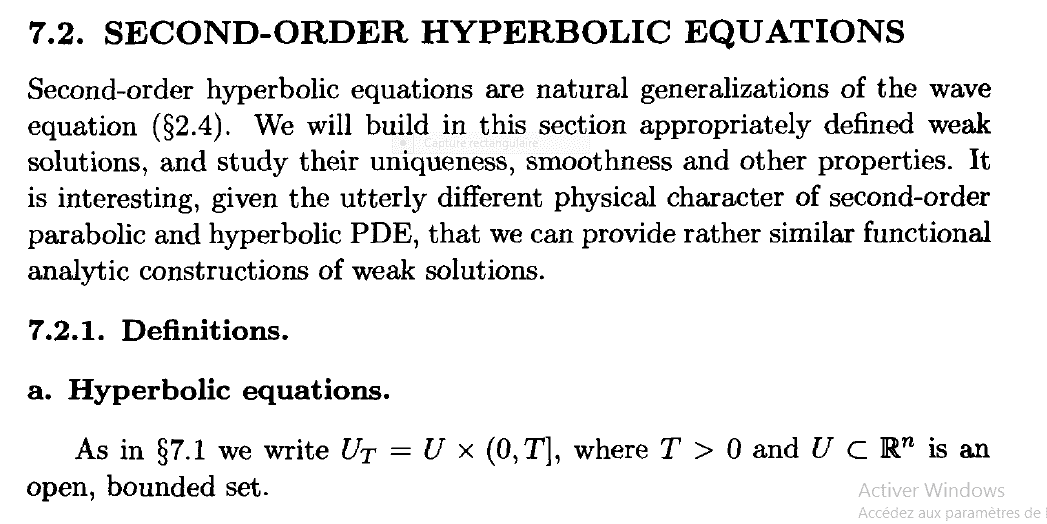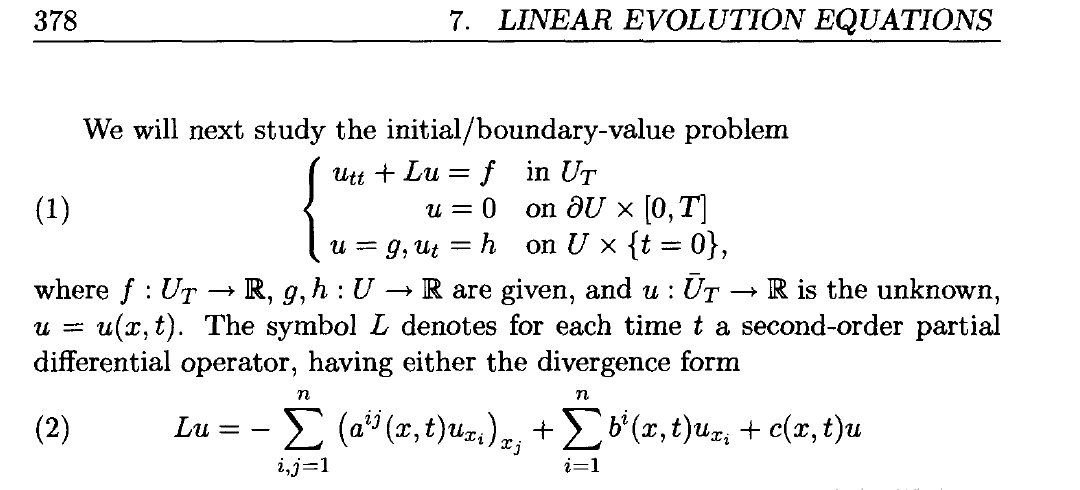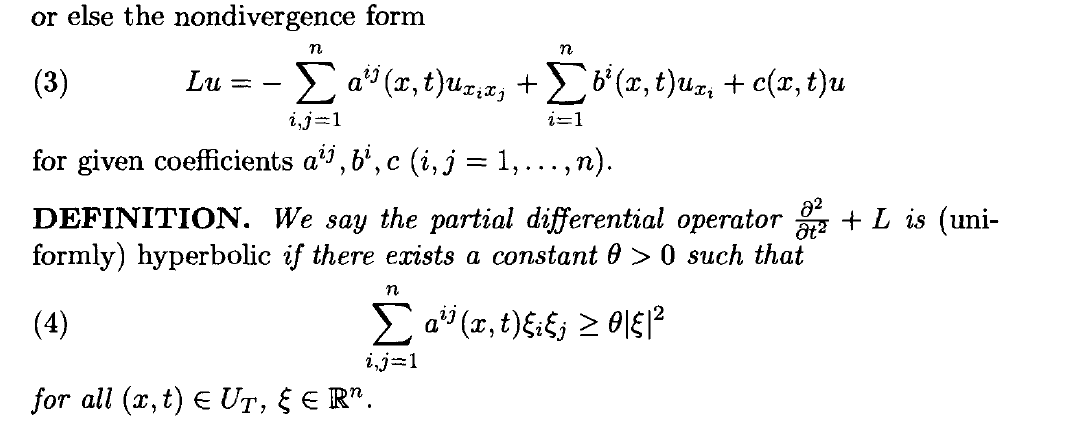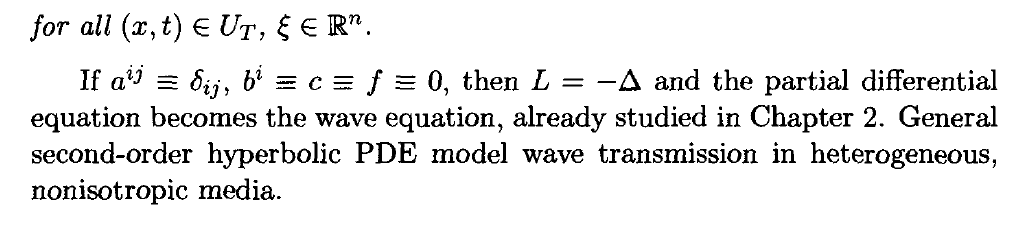# How to prove that the Euler-Bernoulli equation is hyperbolic

• MHB
• mathematix89
I'll let you read it for yourself. -DanI also read a very interesting definition on wikipedia but well...I'll let you read it for yourself. In summary, the following partial differential equation is hyperbolic.f

#### mathematix89

Hello, I would like to prove that the following partial differential equation is hyperbolic. u_{tt} (x,t)+ u_{xxxx} (x,t)= 0 with x \in \left[0 , 1\right] and x \in \left[0 , T \right ] . Can anyone help me? Thank you.

I presume that the last condition is that t, not x, is in [0, T]. Okay, what is the DEFINITION of "hyperbolic partial differential equation"?

I presume that the last condition is that t, not x, is in [0, T]. Okay, what is the DEFINITION of "hyperbolic partial differential equation"?
yes indeed, t \in [0,T]

I presume that the last condition is that t, not x, is in [0, T]. Okay, what is the DEFINITION of "hyperbolic partial differential equation"?
In general when the highest derivative in space and time is of order 2 it is obvious. But here we have a derivative in time of order 2 and in space of order 4.

the definition of these equations that I have in my possession is that given by Lawrence C Evans in his book entitled Partial differential Equations volume 19.
Here is a snippet that gives a definition of these equations (page 377)

In general when the highest derivative in space and time is of order 2 it is obvious. But here we have a derivative in time of order 2 and in space of order 4.

the definition of these equations that I have in my possession is that given by Lawrence C Evans in his book entitled Partial differential Equations volume 19.
Here is a snippet that gives a definition of these equations (page 377)
If we had the book that would be great. If you can't type it out you can write it down on paper (neatly please!) and upload the picture.

-DanIf we had the book that would be great. If you can't type it out you can write it down on paper (neatly please!) and upload the picture.

-Dan
ok I'll try to send you the book or in the worst case I'll take screenshots

ok I'll try to send you the book or in the worst case I'll take screenshotsI also read a very interesting definition on wikipedia but well...